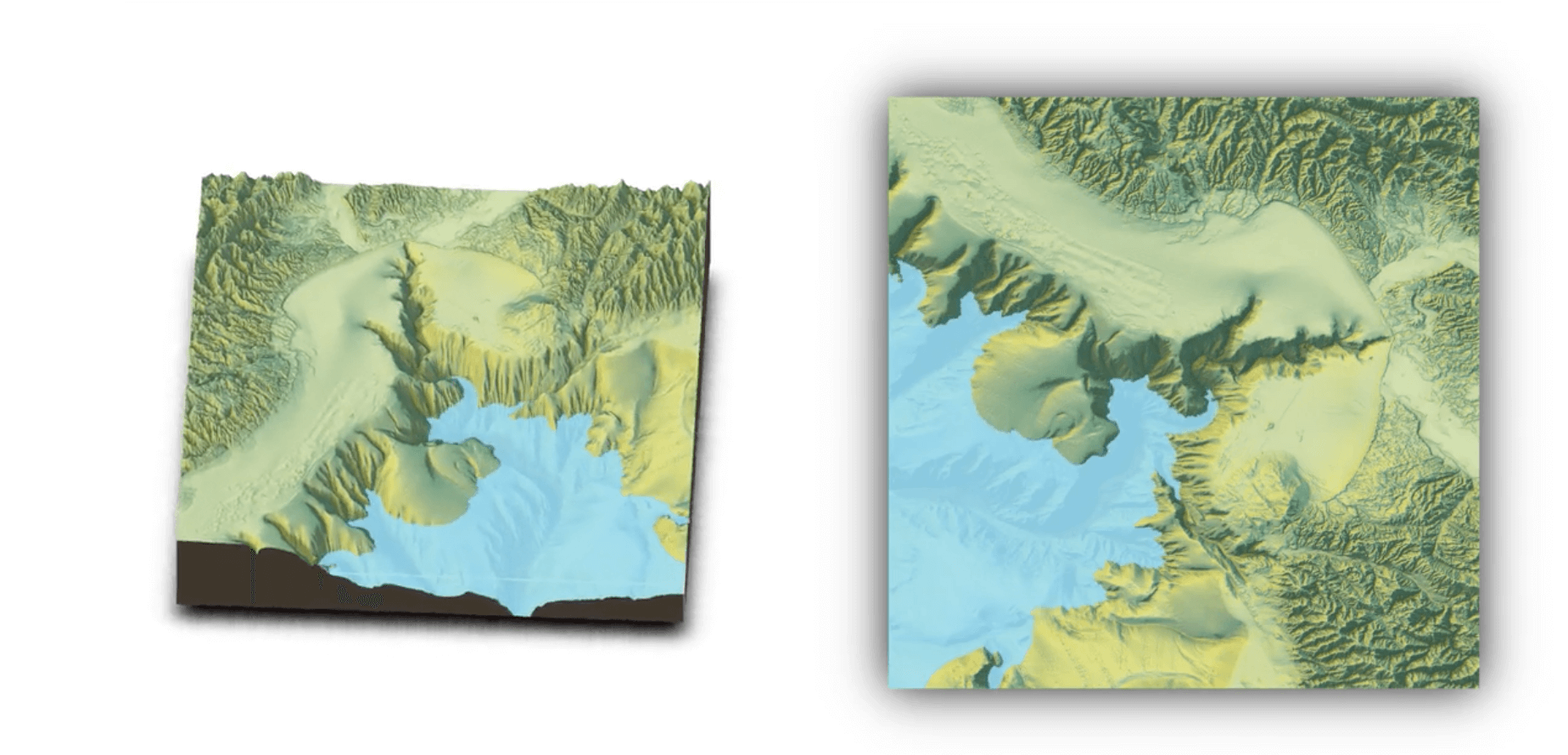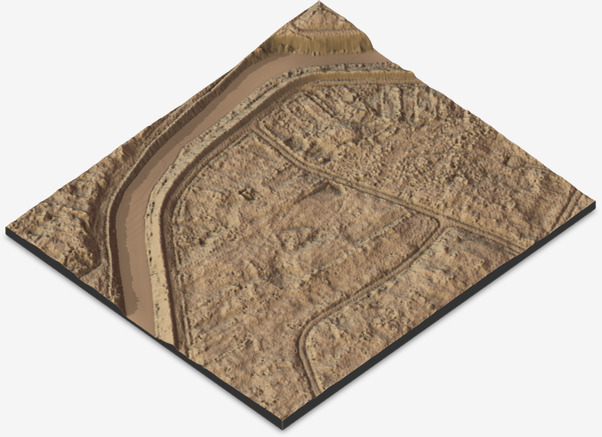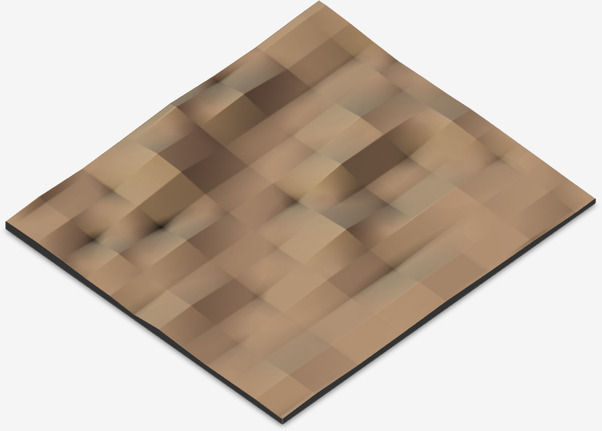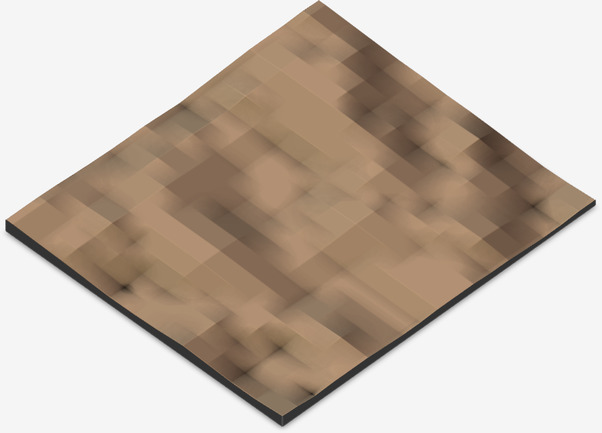rayshader is an amazing R package for producing realistic 3D maps from elevation data. The maps produced by rayshader are much easier to interpret than 2D hillshaded or heatmap images, plus they’re just really cool to look at.From rayshader.com

Here at GPXZ we do our geospatial analysis in Python, but the rayshader package is only available for R and there’s nothing similar for Python. Fortunately it’s possible to call rayshader from Python using rpy2.

## Installation

You’ll need to have rpy2 installed

``````pip install rpy2
``````

and will also need to have `rayshader` installed in R

``````install.packages("devtools")
``````

## Usage

We’ll need to import some standard python geospatial packages, import rpy2, and activate rpy2’s numpy translation layer.

``````import tempfile

import matplotlib.pyplot as plt
import numpy as np
import rasterio as rio
import rpy2.robjects as ro
import rpy2.robjects.numpy2ri
import rpy2.robjects.packages as rpackages

rpy2.robjects.numpy2ri.activate()
``````

I’ll load the same raster used in the rayshader.com getting started example into a 2D numpy array.

``````zip_url = '/vsizip//vsicurl/https://tylermw.com/data/dem_01.tif.zip/dem_01.tif'
with rio.open(zip_url) as f:

plt.imshow(z)
``````The resulting heatmap gives shows the elevation profile, but isn’t super intuitive. That’s where rayshader steps in.

rpy2 has a number of different interfaces, but the easiest thing was to load all the variables into the r namespace, then call the R code as a string.

``````def rayshade(z, img_path=None, zscale=10, fov=0, theta=135, zoom=0.75, phi=45, windowsize=(1000, 1000)):

# Output path.
if not img_path:
img_path = tempfile.NamedTemporaryFile(suffix='.png').name

# Import needed packages.

# Convert array to matrix.
z = np.asarray(z)
rows, cols = z.shape
z_mat = ro.r.matrix(z, nrow=rows, ncol=cols)
ro.globalenv['elmat'] = z_mat

# Save python state to r.
ro.globalenv['img_path'] = img_path
ro.globalenv['zscale'] = zscale
ro.globalenv['fov'] = fov
ro.globalenv['theta'] = theta
ro.globalenv['zoom'] = zoom
ro.globalenv['phi'] = phi
ro.globalenv['windowsize'] = ro.IntVector(windowsize)

# Do the render.
ro.r('''
elmat %>%
plot_3d(elmat, zscale = zscale, fov = fov, theta = theta, zoom = zoom, phi = phi, windowsize = windowsize)
Sys.sleep(0.2)
render_snapshot(img_path)
''')

# Return path.
return img_path
``````

This function returns the path to a rendered 3D png. From there you could copy the file or display it in a jupyter notebook.

``````img_path = rayshade(z)

from IPython.display import Image
Image(filename=img_path)
``````